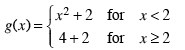### Home > PC > Chapter 2 > Lesson 2.3.1 > Problem2-76

2-76.
1. Define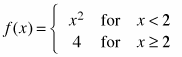. 2-76 HW eTool (Desmos) Homework Help ✎

1. Let g(x) = f(x) + 2 and k(x) = f (x − 3). Sketch the graphs of f(x), g(x), and k(x) on the same set of axes. Use contrasting colors and label the graphs.

2. Write two equations that could represent the functions g(x) and k(x). Watch out for changes in the domain.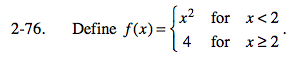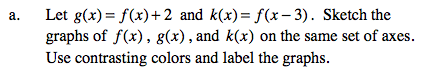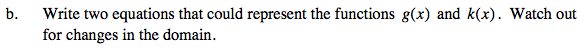Here is one of the functions. Find the other.